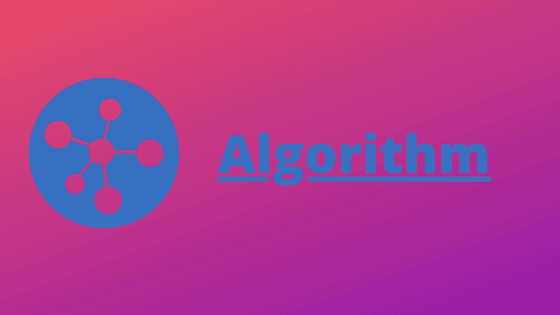# What is an Algorithm explanation with example

Algorithm: In this post, we are going to learn about the term called an algorithm. and we will learn that what is an algorithm and why and where we use the algorithm. and how important it is to solve a particular problem, not a technical problem but also a non-technical problem.## What is an Algorithm?

An algorithm is a step by step method to solving a problem. and in another word, we can say that an algorithm has finite sequence of instructions.

and in order to solve a particular problem, we need a precise and step by step method. not in the term of technology but in our daily life we need a step by step plan to do any work.

### And to obtain an algorithm we need three things.

1. Input
2. a sequence of instructions to process the input.
3. Result

For example, to make a recipe for making potato bondas we need a step by step process like we first need the list of ingredients and then making method and then the expected result will come.

Ingredients
Potatoes 250 grams, chopped onions, finely chopped green chilies, gram flour, oil for frying, water for the batter, salt, etc...

Method
Step 1: Boil potatoes till cooked peel and mash them until they are soft.
Step 2: Mix fried onions, green chilies and salt with the mashed potatoes.
Step 3: Take little portions of the mixture and make small balls.
Step 4: Mix gram flour, water, and a little salt and beat well till a smooth and creamy batter is obtained.
Step 5: Dip the potato balls in the batter. take out and deep fry in oil on a low fire.
Step 6: Take out when the balls are fried to a golden brown color.

Result

In the above example, the Ingredients are work as an input. and the method is working as a sequence of instructions. and then the result comes out as our potato bonds are ready.

### An algorithm has the following five basic characteristics.

First: an algorithm begins with instructions to accept inputs. these inputs are processed by the subsequence and sequence of instructions in the algorithm.
Second: The sequence of instructions specified in the algorithms must be precise and unambiguous. and not be vague.
Third: Each instruction must be sufficiently basic such that it can be carried out by a person with paper and pencil.
Fourth: The total time consumed by the algorithm must be finite.
Five: An algorithm must produce one or more outputs.

let's take another technical example.
A step by step procedure to find the average number of vowels in a passage.

Step 1: Let the number of characters = 0
Step 2: Let number of vowels = 0
Step 3: Repeat Steps 4, 5, 6 and 7 until no more characters are left in the passage.
Step 4: Read one character from the passage.
Step 5: Add 1 to number of characters.
Step 6: If the character is any one of the letters A, E, I, O, U, a, e, i, o, u, add 1 to the number of vowels.
Step 7: Move to the next character.
Step 8: Average Number of vowels = Number of vowels / Number of characters
Step 9: Write the average number of vowels, number of characters.
Step 10: Stop.

#### A process only qualifies as an algorithm. if

• It has Input
• The sequence of instructions is precise.
• The total number of instructions is finite.
• The process need to be terminated on any condition.
• There needs to be output.

and in above process to find the average number of vowels in a passage is an algorithm because it has  number of inputs and the finite number of sequence and instructions and terminate on a condition and have a sufficient output.

Note: So I think now you understand that what is an algorithm and why and where we use it.